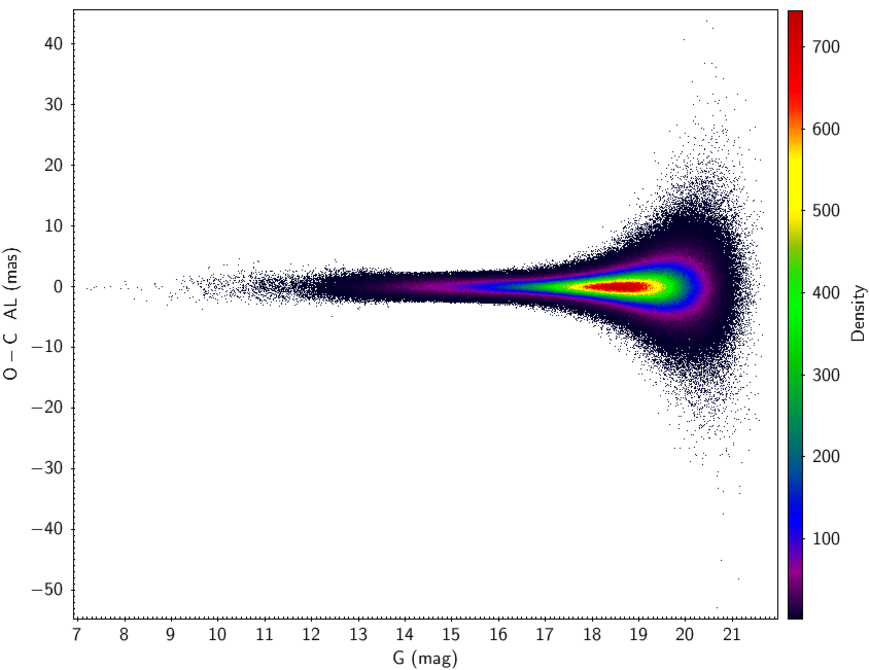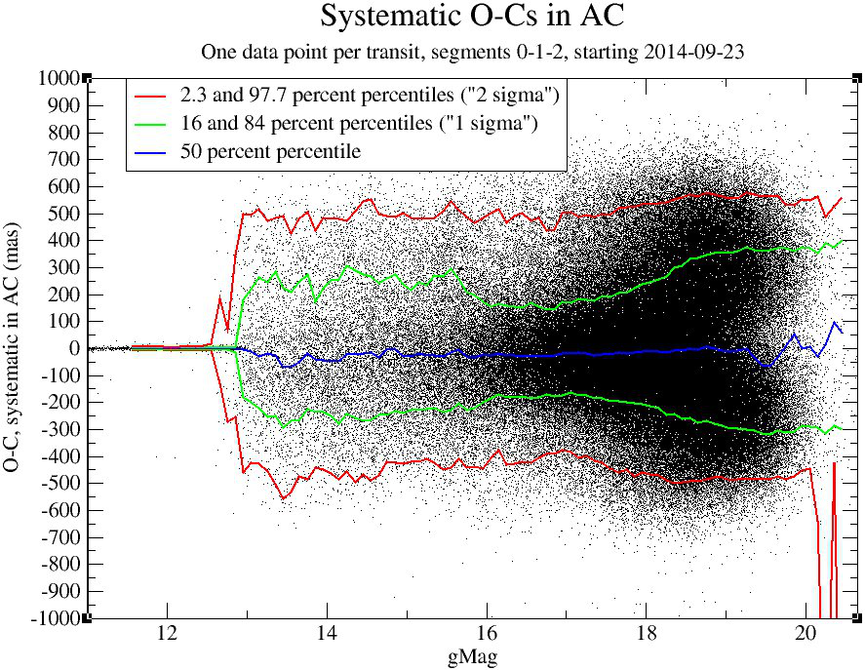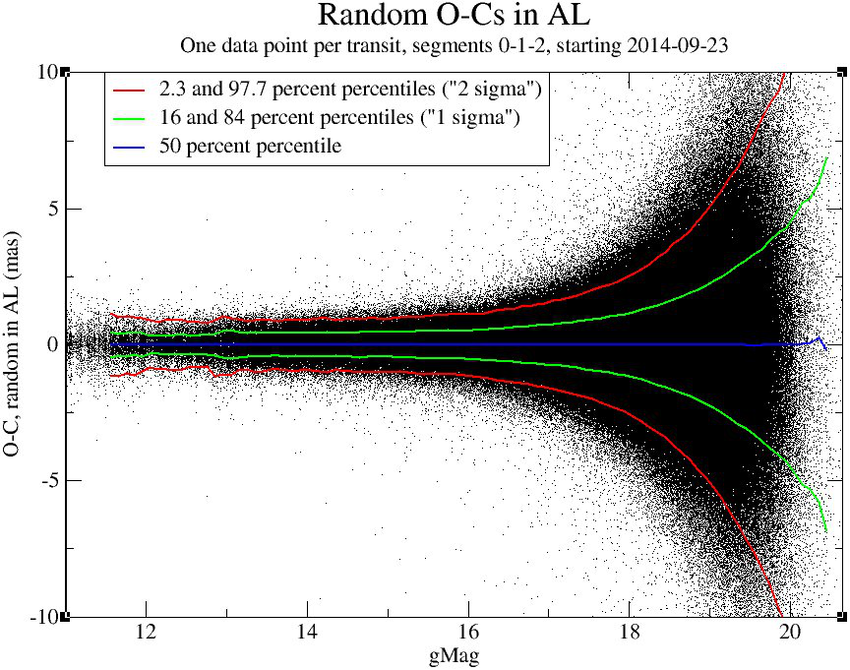# 10.8.2 Validation process

As a result of the orbit determination process, we have rejected 3 objects for which we were not able to fit the Gaia observations to the orbits. These three objects are TNOs with few transits covering a short time span, thus it is not surprising that the orbit determination process fails. The objects are: (2060) Chiron (3 transits, 27 observations), (134340) Pluto (1 transit, 8 observations) and (136199) Eris (2 transits, 15 observations).

Then we have discarded all the outliers produced by the fit (the procedure is explained in Gaia Collaboration et al. 2018f), for a total of $24\,886$ observations and $2\,334$ transits. They are mostly contaminants, such as stars, which have not been removed during the astrometric processing.Figure 10.62: Along scan residuals in function of the G magnitude. For objects fainter than magnitude 10, the outliers are small as expected, but for very bright objects these residuals start to increase and become larger.

Figure 10.62 shows the residuals in the AL direction with respect to the $G$ magnitude, after the outlier rejection procedure. Starting from this result, we have done a more detailed analysis of the residuals, both systematic and random parts, comparing the residuals with the error model produced by the astrometric processing.

We have divided the residuals in their systematic and random part. The systematic part contains the errors which are commons to the whole transit. To obtain the systematic part of the residuals we take the average of the residuals over the complete transit. To obtain the same quantities for the uncertainties, we sum the systematic covariance matrix to the sum of the random components of each CCD, where we have divided only the AL part by the number of observations (because the AL part is the only one that is really random). Then we compare the uncertainties in AL and in AC to the residuals.Figure 10.63: The left panel shows a zoom in the interval [-10, 10] of the systematic part of the AL residuals, that is the average of the residuals in AL over a complete transit, with respect to magnitude values larger than 11. The right panel shows a zoom in the interval [-3, 3] of the systematic part of the AL residuals divided by the expected covariance computed as explained above.

Figure 10.63 shows the systematic part of the AL residuals computed as the average of the residuals in AL over a complete transit (left panel), and the same quantity divided by the expected covariance computed as explained above (right panel). The different lines represent the different percentiles (as in the legend). This figure shows a magnitude dependence of the residuals, which are also increasing for faint objects. It is also clear that in general the residuals are smaller than expected and at its best, around magnitude 16, they are even 10 times smaller than expected.Figure 10.64: The left panel shows a zoom in the [-1000, 1000] interval of the systematic part of the AC residuals, that is the average of the residuals in AL over a complete transit, with respect to magnitude values larger than 11. The right panel shows a zoom in the interval [-3, 3] of the systematic part of the AC residuals divided by the expected covariance computed as explained above.

Figure 10.64 shows the systematic part of the AC residuals and the same quantity divided by the expected covariance, computed as in Figure 10.63. As for the AL component, we find that the residuals behave better than expected.

The result is that the positions reach the expected quality, while the error model needs to be improved, especially in AC where the errors are not Gaussian.

We can do a similar comparison in the random component of the residuals. We take the residuals coming from the validation process and, for each residual, we subtract the mean averaged over the complete transit. The results are showed Figure 10.65 and Figure 10.66. We see that for faint objects the AL component of the residuals increase with magnitude, because the residual is dominated by the centroiding error. For brighter objects the residuals are roughly constant, because they are dominated by the scatter on the attitude, as expected (left panel, Figure 10.65). The scatter of the AL components of the positions inside each transit also really behaves as expected (right panel, Figure 10.65).

Figure 10.66 shows that also the AC residuals are as expected: for the 2-D window (brighter than $G=13$) they are much smaller than for 1-D window, and still smaller than expected for faint objects.

Globally the positions are very good and the error model for the centroiding error as well. As for the systematic part, the error model in the AC direction for the 1-D window can be improved.Figure 10.65: The left panel shows a zoom in the interval [-10, 10] of the random part of the AL residuals, with respect to magnitude values larger than 11. The right panel shows a zoom in the interval [-4, 4] of the random part of the AL residuals divided by their uncertainties.Figure 10.66: The left panel shows a zoom in the interval [-500, 500] of the random part of the AC residuals, with respect to the magnitude values larger than 11. The right panel shows a zoom in the interval [-4, 4] of the random part of the AC residuals divided by their uncertainties.

Objects brighter than magnitude 10 requires a different analysis, because the residuals are much larger than the predicted uncertainties, as is clear Figure 10.67.Figure 10.67: The left panel shows the systematic part of the AL component of the residuals, zoomed in for brighter objects. The right panel shows the same for the random part of the residuals.

The left panel of Figure 10.67 shows that for objects fainter than $G=11$, the systematic part of the residuals is really smaller than expected, but for very bright objects the residuals start to increase and become much larger than expected. The two horizontal lines represent the $2\sigma$ lines between which we expect to find $95\%$ of the data points, and the red dots are the residuals for (1) Ceres.

The right panel of Figure 10.67 shows that up to magnitude 10 the residuals behave as expected, but for brighter objects the random part of the residuals starts to increase. The red dots represent (1) Ceres, and we can see that in this case the random component is almost 5 times larger than expected.

As a result of this analysis, we see that for the objects brighter than magnitude 10 the residuals are much larger than expected and with a percentage of outliers that is much higher than for faint objects. Thus, we have also decided to reject 22 objects, which have at least one transit fainter than magnitude 10. The magnitude has been computed from the fluxes, when available for a given transit, using the formula as in (Riello et al. 2018).

We rejected $27\,981$ observations in total, which correspond to the observations belonging to the 25 rejected objects and the $24\,886$ outliers.